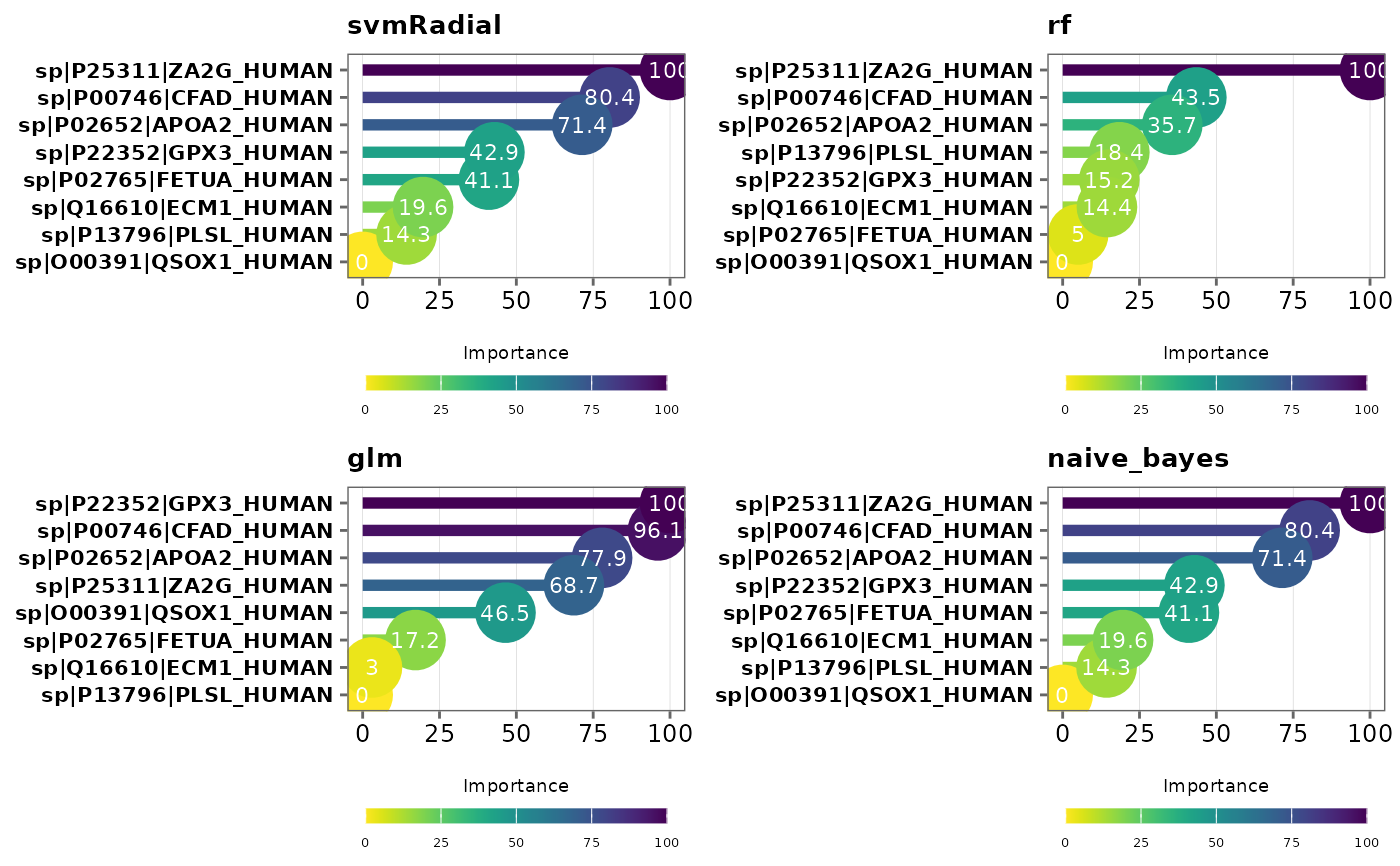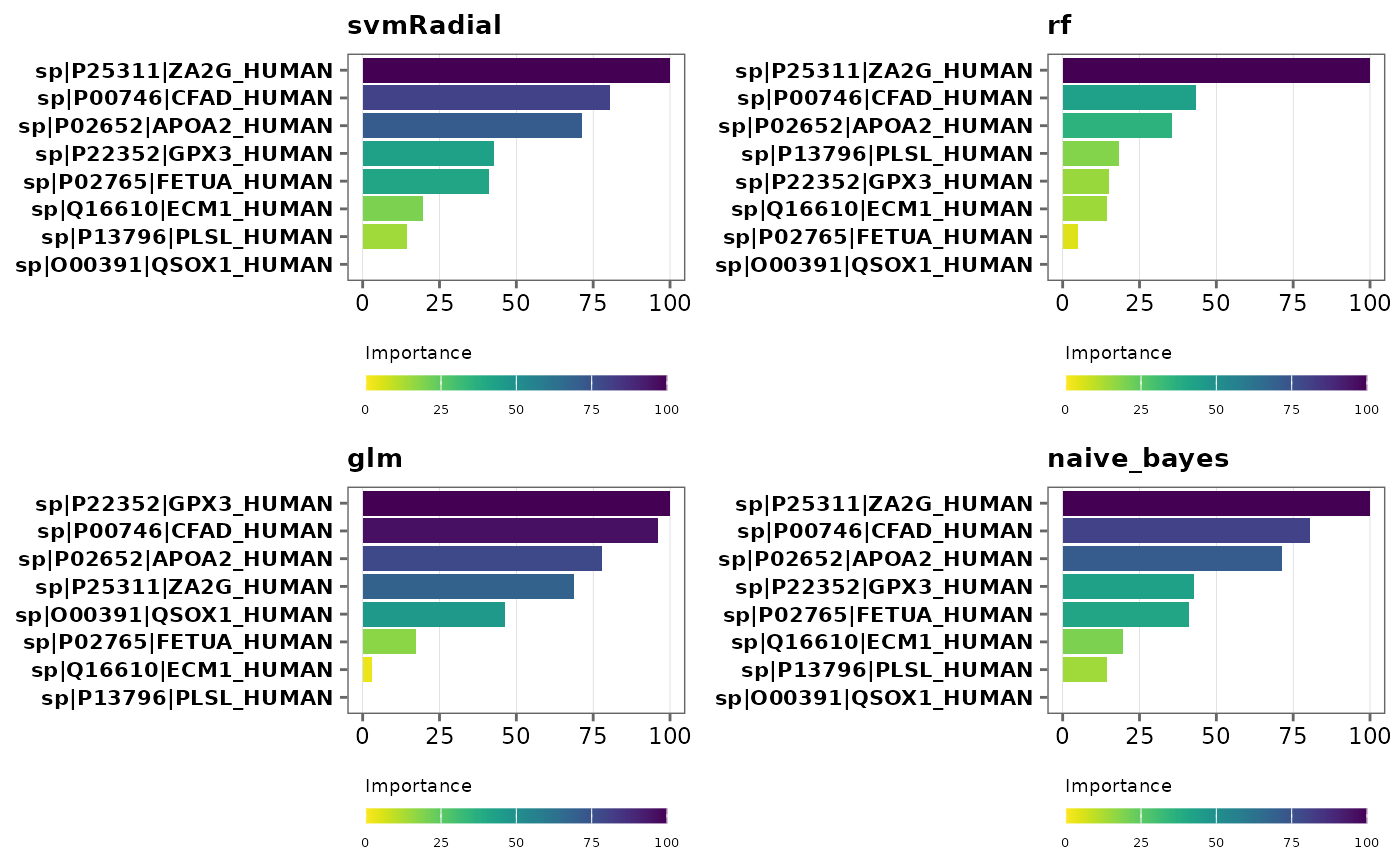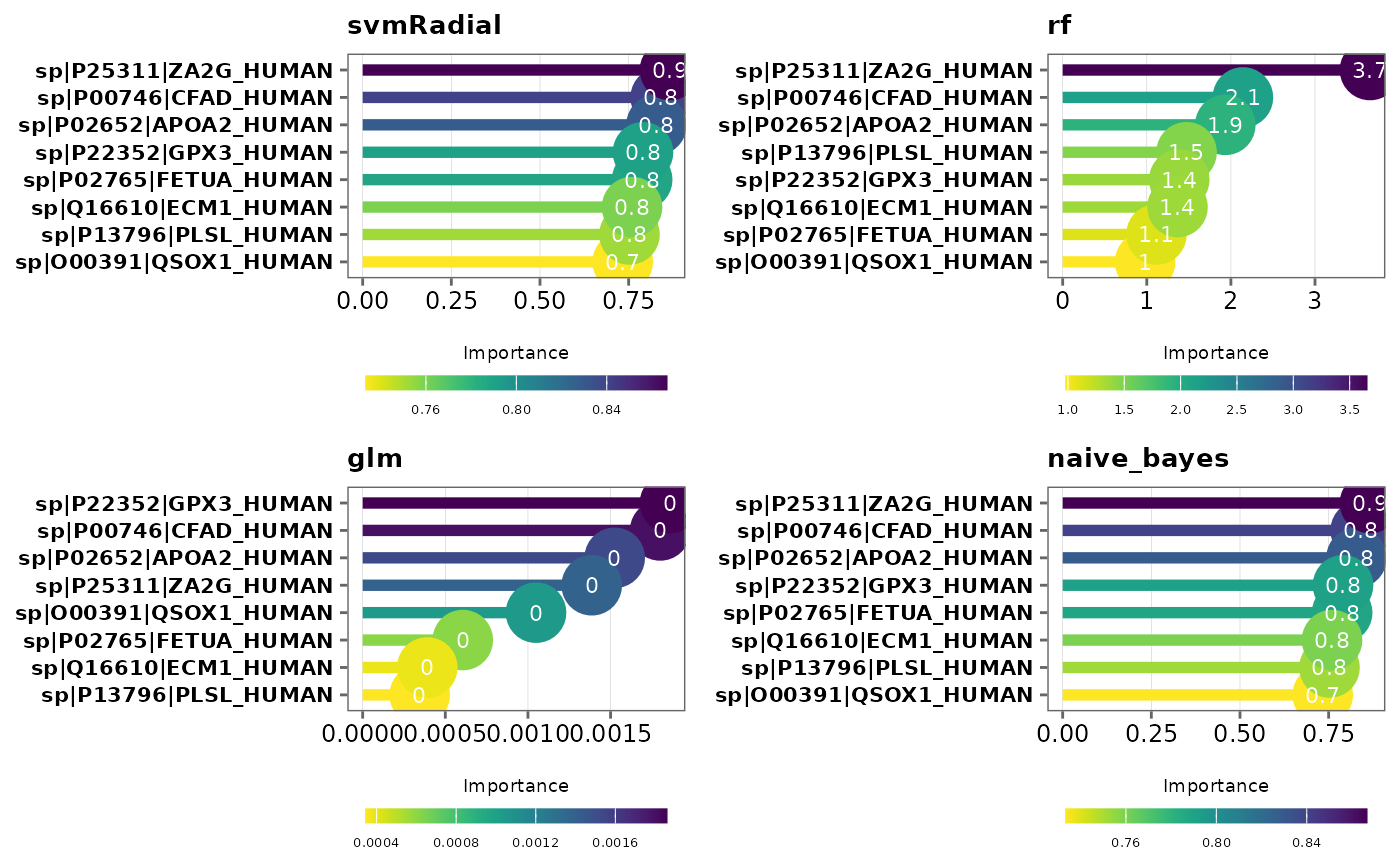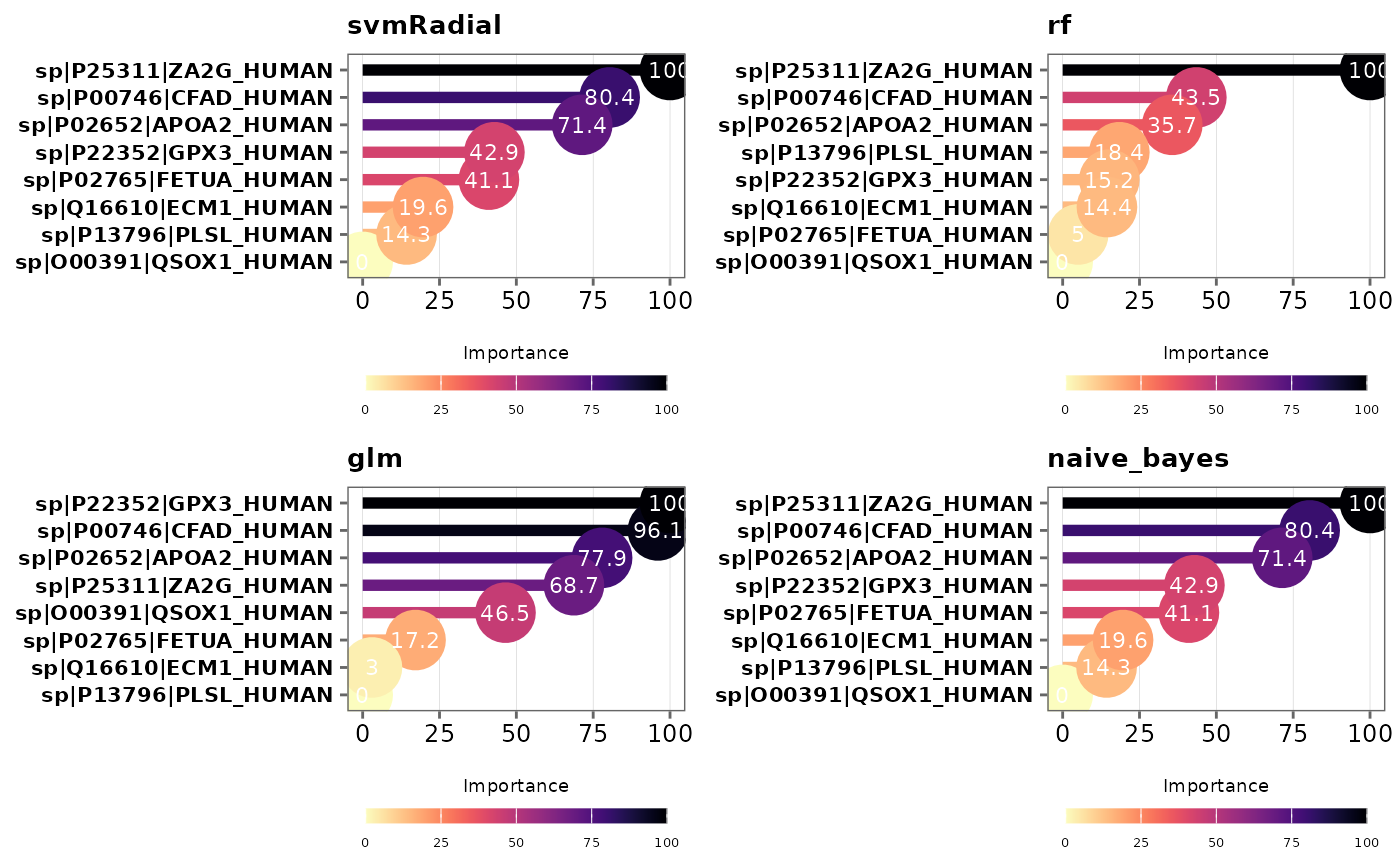This function visualizes variable importance in models

## Usage

varimp_plot(
model_list,
...,
type = "lollipop",
text_size = 10,
palette = "viridis",
n_row,
n_col,
save = FALSE,
file_path = NULL,
file_name = "VarImp_plot",
file_type = "pdf",
dpi = 80,
plot_width = 7,
plot_height = 7
)

## Arguments

model_list

A model_list object from performing train_models.

...

Additional arguments to be passed on to varImp.

type

Type of plot to generate. Choices are "bar" or "lollipop." Default is "lollipop."

text_size

Text size for plot labels, axis labels etc. Default is 10.

palette

Viridis color palette option for plots. Default is "viridis". See viridis for available options.

n_row

Number of rows to print the plots.

n_col

Number of columns to print the plots.

save

Logical. If TRUE saves a copy of the plot in the directory provided in file_path.

file_path

A string containing the directory path to save the file.

file_name

File name to save the plot. Default is "VarImp_plot."

file_type

File type to save the plot. Default is "pdf".

dpi

Plot resolution. Default is 80.

plot_width

Width of the plot. Default is 7.

plot_height

Height of the plot. Default is 7.

## Value

A list of ggplot2 objects.

## Details

• Note: Variables are ordered by variable importance in descending order, and by default, importance values are scaled to 0 and 100. This can be changed by specifying scale = FALSE. See varImp for more information.

• train_models, rem_feature

• varImp

## Author

Chathurani Ranathunge

## Examples

# \donttest{

## Create a model_df object
covid_model_df <- pre_process(covid_fit_df, covid_norm_df)
#> Total number of differentially expressed proteins (8) is less than n_top.
#> None of the proteins show high pair-wise correlation.
#>
#> No highly correlated proteins to be removed.

## Split the data frame into training and test data sets
covid_split_df <- split_data(covid_model_df)

## Fit models based on the default list of machine learning (ML) algorithms
covid_model_list <- train_models(covid_split_df)
#>
#>
#> Running rf...
#>
#> Running glm...
#> Warning: glm.fit: algorithm did not converge
#> Warning: glm.fit: fitted probabilities numerically 0 or 1 occurred
#> Warning: glm.fit: algorithm did not converge
#> Warning: glm.fit: fitted probabilities numerically 0 or 1 occurred
#> Warning: glm.fit: algorithm did not converge
#> Warning: glm.fit: fitted probabilities numerically 0 or 1 occurred
#> Warning: glm.fit: algorithm did not converge
#> Warning: glm.fit: fitted probabilities numerically 0 or 1 occurred
#> Warning: glm.fit: algorithm did not converge
#> Warning: glm.fit: fitted probabilities numerically 0 or 1 occurred
#> Warning: glm.fit: algorithm did not converge
#> Warning: glm.fit: fitted probabilities numerically 0 or 1 occurred
#> Warning: glm.fit: algorithm did not converge
#> Warning: glm.fit: fitted probabilities numerically 0 or 1 occurred
#> Warning: glm.fit: algorithm did not converge
#> Warning: glm.fit: fitted probabilities numerically 0 or 1 occurred
#> Warning: glm.fit: algorithm did not converge
#> Warning: glm.fit: fitted probabilities numerically 0 or 1 occurred
#> Warning: glm.fit: algorithm did not converge
#> Warning: glm.fit: fitted probabilities numerically 0 or 1 occurred
#> Warning: glm.fit: algorithm did not converge
#> Warning: glm.fit: fitted probabilities numerically 0 or 1 occurred
#> Warning: glm.fit: algorithm did not converge
#> Warning: glm.fit: fitted probabilities numerically 0 or 1 occurred
#> Warning: glm.fit: algorithm did not converge
#> Warning: glm.fit: fitted probabilities numerically 0 or 1 occurred
#> Warning: glm.fit: algorithm did not converge
#> Warning: glm.fit: fitted probabilities numerically 0 or 1 occurred
#> Warning: glm.fit: algorithm did not converge
#> Warning: glm.fit: fitted probabilities numerically 0 or 1 occurred
#> Warning: glm.fit: algorithm did not converge
#> Warning: glm.fit: fitted probabilities numerically 0 or 1 occurred
#> Warning: glm.fit: algorithm did not converge
#> Warning: glm.fit: fitted probabilities numerically 0 or 1 occurred
#> Warning: glm.fit: algorithm did not converge
#> Warning: glm.fit: fitted probabilities numerically 0 or 1 occurred
#> Warning: glm.fit: algorithm did not converge
#> Warning: glm.fit: fitted probabilities numerically 0 or 1 occurred
#> Warning: glm.fit: algorithm did not converge
#> Warning: glm.fit: fitted probabilities numerically 0 or 1 occurred
#> Warning: glm.fit: algorithm did not converge
#> Warning: glm.fit: fitted probabilities numerically 0 or 1 occurred
#> Warning: glm.fit: algorithm did not converge
#> Warning: glm.fit: fitted probabilities numerically 0 or 1 occurred
#> Warning: glm.fit: algorithm did not converge
#> Warning: glm.fit: fitted probabilities numerically 0 or 1 occurred
#> Warning: glm.fit: algorithm did not converge
#> Warning: glm.fit: fitted probabilities numerically 0 or 1 occurred
#> Warning: glm.fit: algorithm did not converge
#> Warning: glm.fit: fitted probabilities numerically 0 or 1 occurred
#> Warning: glm.fit: algorithm did not converge
#> Warning: glm.fit: fitted probabilities numerically 0 or 1 occurred
#> Warning: glm.fit: algorithm did not converge
#> Warning: glm.fit: fitted probabilities numerically 0 or 1 occurred
#> Warning: glm.fit: algorithm did not converge
#> Warning: glm.fit: fitted probabilities numerically 0 or 1 occurred
#> Warning: glm.fit: algorithm did not converge
#> Warning: glm.fit: fitted probabilities numerically 0 or 1 occurred
#> Warning: glm.fit: algorithm did not converge
#> Warning: glm.fit: fitted probabilities numerically 0 or 1 occurred
#> Warning: glm.fit: algorithm did not converge
#> Warning: glm.fit: fitted probabilities numerically 0 or 1 occurred
#>
#> Running xgbLinear...
#>
#> Running naive_bayes...
#> Done!

## Variable importance - lollipop plots
varimp_plot(covid_model_list)## Bar plots
varimp_plot(covid_model_list, type = "bar")## Do not scale variable importance values
varimp_plot(covid_model_list, scale = FALSE)## Change color palette
varimp_plot(covid_model_list, palette = "magma")# }